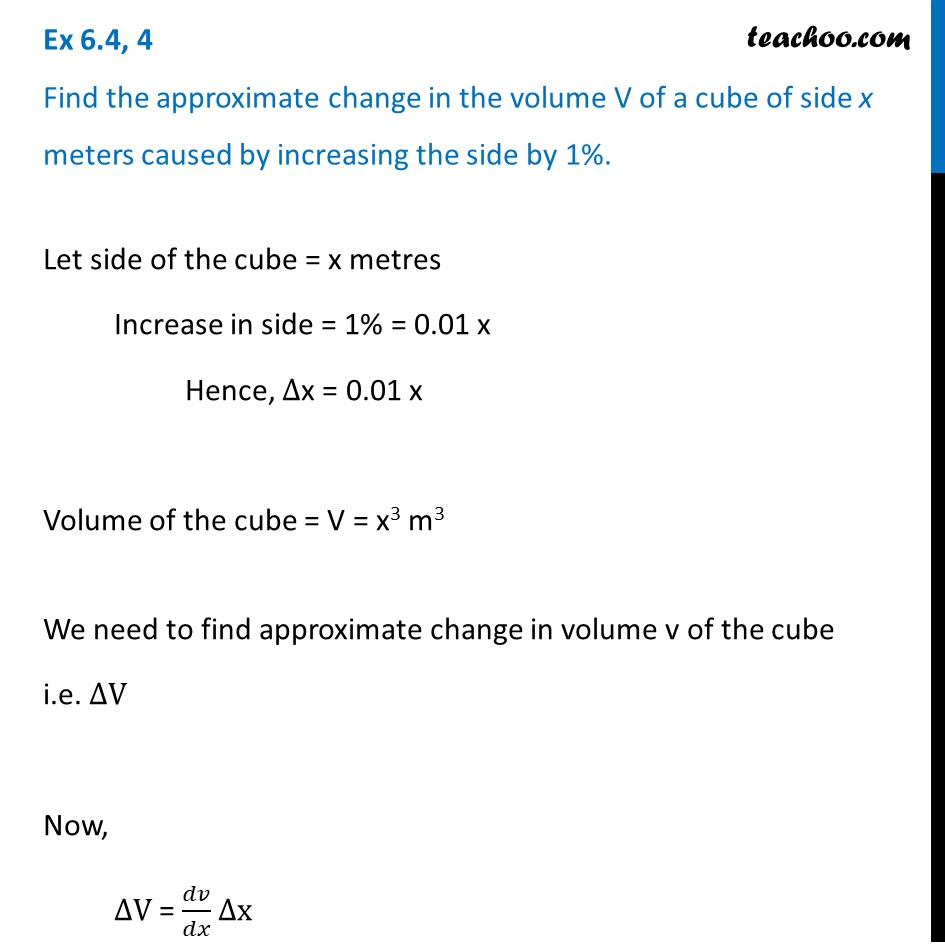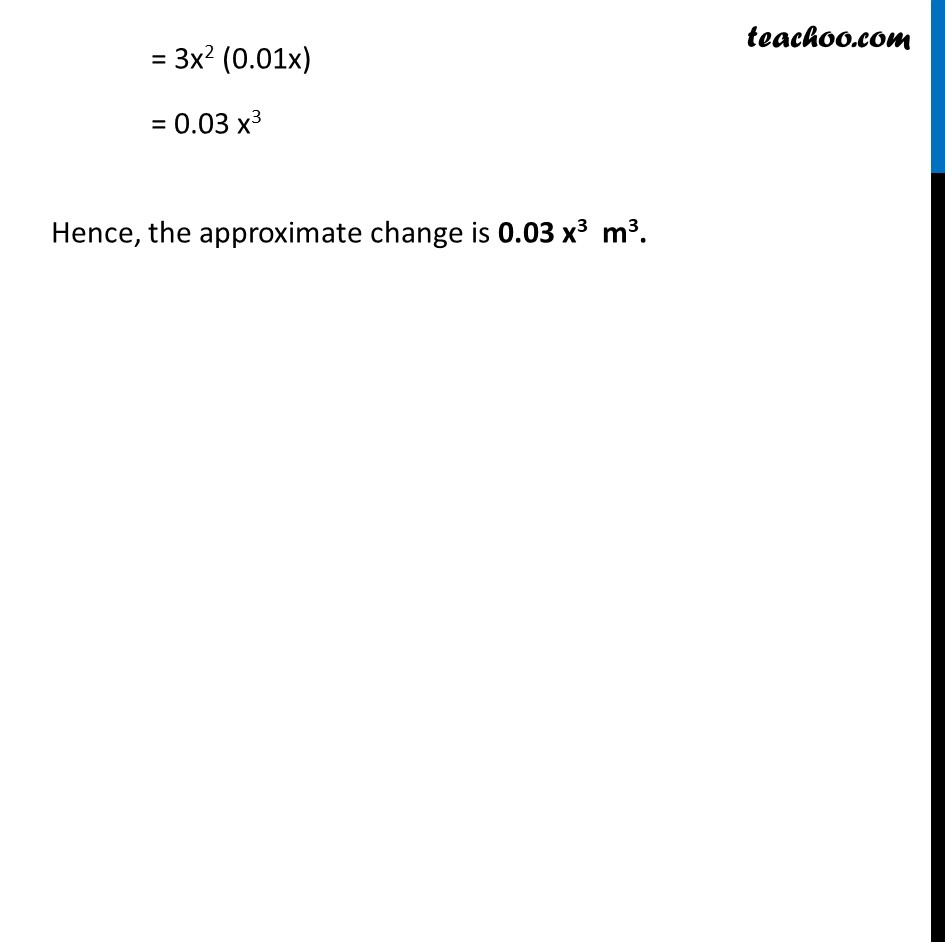Approximations (using Differentiation)

Chapter 6 Class 12 Application of Derivatives
Serial order wiseLearn in your speed, with individual attention - Teachoo Maths 1-on-1 Class

### Transcript

Question 4 Find the approximate change in the volume V of a cube of side x meters caused by increasing the side by 1%.Let side of the cube = x metres Increase in side = 1% = 0.01 x Hence, ∆x = 0.01 x Volume of the cube = V = x3 m3 We need to find approximate change in volume v of the cube i.e. ∆V Now, ∆V = 𝑑𝑣/𝑑𝑥 ∆x = (𝑑(𝑥^3))/𝑑𝑥 ∆x = 3x2 (0.01x) = 0.03 x3 Hence, the approximate change is 0.03 x3 m3.